# Practical Benefits:Negative Feedback

Most practical amplifier circuits use negative feedback for the following practical benefits:

 Stabilization of voltage gain Decreasing output impedance Increasing input impedance Decreasing distortion Increasing bandwidth
Index

Electronics concepts

Op-amp concepts

Feedback concepts

 HyperPhysics*****Electricity and magnetism R Nave
Go Back

# Stabilization of Voltage Gain

One of the benefits of negative feedback is the stabilization of the voltage gain of an amplifier against changes in the components (e.g., with temperature, frequency, etc. ). If you represent the gain without feedback (the open loop gain) by A0 , then the system gain with negative feedback is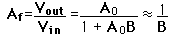where B is the fraction of the output which feeds back as a negative voltage at the input. The extent of this stabilizing influence can be illustrated as follows: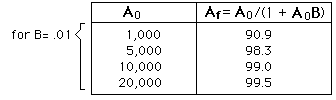This stabilization increases the effective bandwidth.
 Why use negative feedback?
Index

Electronics concepts

Op-amp concepts

Feedback concepts

 HyperPhysics*****Electricity and magnetism R Nave
Go Back

# Increasing Input Impedance

 The input impedance is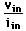which without negative feedback is Zin0 .
 But with feedback, the current is reduced to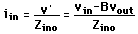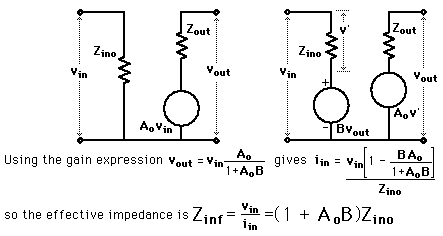Why use negative feedback?
Index

Electronics concepts

Feedback concepts

Reference
Simpson
Sec 7.2

 HyperPhysics*****Electricity and magnetism R Nave
Go Back

# Decreasing Output Impedance

As in the approach to input impedance, the effect of negative feedback on output impedance can be obtained by analysis of the equivalent circuit.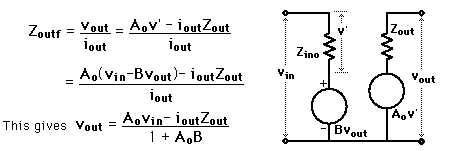Things become more subtle here because the input voltage vin must be held constant while we see how vout varies with iout . The easiest way to do this is with the partial derivative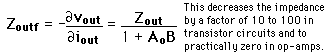Why use negative feedback?
Index

Electronics concepts

Feedback concepts

Reference
Simpson
Sec 7.2

 HyperPhysics*****Electricity and magnetism R Nave
Go Back